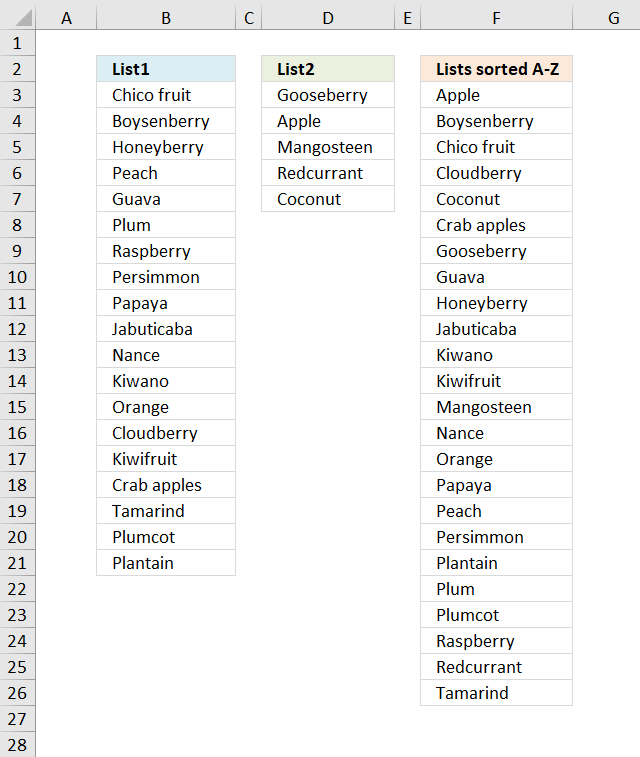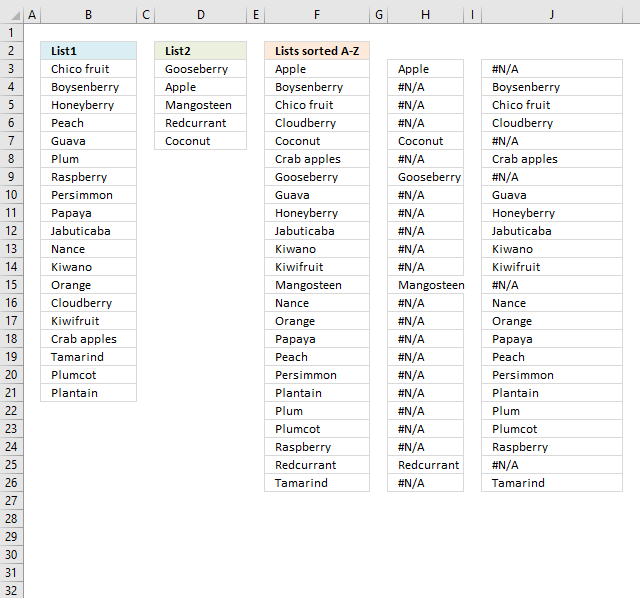Author: Oscar Cronquist Article last updated on November 21, 2018Array formula in cell F3:

=IFERROR(INDEX(\$D\$3:\$D\$7, MATCH(ROWS(F\$3:\$F3)-1, COUNTIF(\$D\$3:\$D\$7, "<"&\$D\$3:\$D\$7)+COUNTIF(\$B\$3:\$B\$21, "<"&\$D\$3:\$D\$7)+IF((COUNTIF(\$D\$3:\$D\$7, \$D\$3:\$D\$7)+COUNTIF(\$B\$3:\$B\$21, \$D\$3:\$D\$7))<=COUNTIF(\$F\$2:F2, \$D\$3:\$D\$7), 0, COUNTIF(\$F\$2:F2, \$D\$3:\$D\$7)), 0)), INDEX(\$B\$3:\$B\$21, MATCH(ROWS(F\$3:\$F3)-1, COUNTIF(\$B\$3:\$B\$21, "<"&\$B\$3:\$B\$21)+COUNTIF(\$D\$3:\$D\$7, "<"&\$B\$3:\$B\$21)+IF((COUNTIF(\$B\$3:\$B\$21, \$B\$3:\$B\$21)+COUNTIF(\$D\$3:\$D\$7, \$B\$3:\$B\$21))<=COUNTIF(\$F\$2:F2, \$B\$3:\$B\$21), 0, COUNTIF(\$F\$2:F2, \$B\$3:\$B\$21)), 0)))

This array formula handles duplicates but not blank cells.

### How to create an array formula

1. Select cell F3
2. Copy/Paste above formula
3. Press and hold Ctrl + Shift
4. Press enter once
5. Release all keys

### How to copy array formula

1. Select cell F3.
2. Copy cell (Ctrl + c).
3. Paste to cells below (Ctrl + v).

### Explaining formula in cell F3

The IFERROR function makes the formula alternate between two lists depending on the sort order of each value.

IFERROR(part1, part2)

#### Step 1 - Build expanding number

Every value in these two lists will have number which corresponds to the sort order. To match each value we need to build a formula that returns a number from 0 (zero) to whatever.

The ROWS function returns a number based on an expanding cell reference, it grows automatically as the cell is copied to cells below.

ROWS(F\$3:\$F3)-1

becomes

1-1 and returns 0 (zero).

#### Step 2 - Create an array that shows the sort order of each value as if they were sorted alphabetically.

The COUNTIF function counts values based on a condition or criteria. If we add a "<" the function returns a value that shows the rank if the list were sorted. The ampersand concatenates the "<" with the values in the second argument.

COUNTIF(\$D\$3:\$D\$7, "<"&\$D\$3:\$D\$7)

becomes

COUNTIF({"Gooseberry";"Apple";"Mangosteen";"Redcurrant";"Coconut"}, "<"&{"Gooseberry";"Apple";"Mangosteen";"Redcurrant";"Coconut"})

becomes

COUNTIF({"Gooseberry";"Apple";"Mangosteen";"Redcurrant";"Coconut"}, {"<Gooseberry";"<Apple";"<Mangosteen";"<Redcurrant";"<Coconut"})

and returns

{2;0;3;4;1}.

For example, how many values are sorted above "Apple" in array {"Gooseberry";"Apple";"Mangosteen";"Redcurrant";"Coconut"}? None, so Apple gets number 0 (zero).

#### Step 3 - Compare values to the other list

COUNTIF(\$B\$3:\$B\$21, "<"&\$D\$3:\$D\$7)

becomes

COUNTIF({"Chico fruit";"Boysenberry";"Honeyberry";"Peach";"Guava";"Plum";"Raspberry";"Persimmon";"Papaya";"Jabuticaba";"Nance";"Kiwano";"Orange";"Cloudberry";"Kiwifruit";"Crab apples";"Tamarind";"Plumcot";"Plantain"}, "<"&\$D\$3:\$D\$7)

and returns

{4;0;9;18;3}.

Apple is clearly the top value if these values are combined and sorted from A to Z since this array also says that "Apple" is 0 (zero).

#### Step 4 - Take duplicates into account

This step adds 1 to the correct value in the array so also duplicates in both lists are returned. The IF function makes sure that previously counted values are taken into account.

IF((COUNTIF(\$D\$3:\$D\$7, \$D\$3:\$D\$7)+COUNTIF(\$B\$3:\$B\$21, \$D\$3:\$D\$7))<=COUNTIF(\$F\$2:F2, \$D\$3:\$D\$7), 0, COUNTIF(\$F\$2:F2, \$D\$3:\$D\$7))

becomes

IF(({1;1;1;1;1}+{0;0;0;0;0})<=COUNTIF(\$F\$2:F2, \$D\$3:\$D\$7), 0, COUNTIF(\$F\$2:F2, \$D\$3:\$D\$7))

becomes

IF(({1;1;1;1;1}+{0;0;0;0;0})<={0;0;0;0;0}, 0, {0;0;0;0;0})

becomes

IF({1;1;1;1;1}<={0;0;0;0;0}, 0, {0;0;0;0;0})

becomes

IF({FALSE;FALSE;FALSE;FALSE;FALSE}, 0, {0;0;0;0;0})

and returns

{0;0;0;0;0}

#### Step 5 - Add arrays

COUNTIF(\$D\$3:\$D\$7, "<"&\$D\$3:\$D\$7)+COUNTIF(\$B\$3:\$B\$21, "<"&\$D\$3:\$D\$7)+IF((COUNTIF(\$D\$3:\$D\$7, \$D\$3:\$D\$7)+COUNTIF(\$B\$3:\$B\$21, \$D\$3:\$D\$7))<=COUNTIF(\$F\$2:F2, \$D\$3:\$D\$7), 0, COUNTIF(\$F\$2:F2, \$D\$3:\$D\$7))

becomes

{2;0;3;4;1} + {4;0;9;18;3} + {0;0;0;0;0}

and returns

{6;0;12;22;4}

#### Step 6 - Match number to array

The MATCH function returns the relative position of a given value in a cell range or array.

MATCH(ROWS(F\$3:\$F3)-1, COUNTIF(\$B\$3:\$B\$21, "<"&\$B\$3:\$B\$21)+COUNTIF(\$D\$3:\$D\$7, "<"&\$B\$3:\$B\$21)+IF((COUNTIF(\$B\$3:\$B\$21, \$B\$3:\$B\$21)+COUNTIF(\$D\$3:\$D\$7, \$B\$3:\$B\$21))<=COUNTIF(\$F\$2:F2, \$B\$3:\$B\$21), 0, COUNTIF(\$F\$2:F2, \$B\$3:\$B\$21)), 0)

becomes

MATCH(0, COUNTIF(\$B\$3:\$B\$21, "<"&\$B\$3:\$B\$21)+COUNTIF(\$D\$3:\$D\$7, "<"&\$B\$3:\$B\$21)+IF((COUNTIF(\$B\$3:\$B\$21, \$B\$3:\$B\$21)+COUNTIF(\$D\$3:\$D\$7, \$B\$3:\$B\$21))<=COUNTIF(\$F\$2:F2, \$B\$3:\$B\$21), 0, COUNTIF(\$F\$2:F2, \$B\$3:\$B\$21)), 0)

becomes

MATCH(0, {6;0;12;22;4}, 0)

and returns 2.

#### Step 7 - Return value based on position

INDEX(\$D\$3:\$D\$7, MATCH(ROWS(F\$3:\$F3)-1, COUNTIF(\$D\$3:\$D\$7, "<"&\$D\$3:\$D\$7)+COUNTIF(\$B\$3:\$B\$21, "<"&\$D\$3:\$D\$7)+IF((COUNTIF(\$D\$3:\$D\$7, \$D\$3:\$D\$7)+COUNTIF(\$B\$3:\$B\$21, \$D\$3:\$D\$7))<=COUNTIF(\$F\$2:F2, \$D\$3:\$D\$7), 0, COUNTIF(\$F\$2:F2, \$D\$3:\$D\$7)), 0))

becomes

INDEX(\$D\$3:\$D\$7, 2)

and returns

"Apple" in cell F3.

If an error had been returned the IFERROR function then continues with the second part of the formula. It works just the same as the first part, however, it returns values based on List1.

The following image shows what the two parts return, the first part of the formula in column H and the second part in column J.sort-from-a-to-z-from-two-columns.xlsx

This blog article is one out of five articles on the same subject:

Sort a column alphabetically

Table of Contents Sort a column using array formula Two columns sorting by the second column Sort alphanumeric values I […]

Populate drop down list with unique distinct values sorted from A to Z

Question: How do I create a drop-down list with unique distinct alphabetically sorted values? Table of contents Sort values using […]

Sort a range from A to Z [Array formula]

Question: How do I sort a range alphabetically using excel array formula? Answer: Cell range \$B\$2:\$E\$5 contains text values in random […]

### Sort text from multiple cell ranges combined (user defined function)This user defined function allows you to enter up to 255 arguments or cell ranges. The udf combines all values from all cell ranges and then sorts them from A to Z. It uses a bubblesort algorithm and I don't recommend using large data sets.

Array formula in cell F2:F12:

=SortMultipleRanges(A2:B3,C4:C6,D8:D9)

VBA Code

```Function SortMultipleRanges(ParamArray rng() As Variant)
Dim temp() As Variant

'Count cells
For Each cellrange In rng
i = i + cellrange.Cells.CountLarge
Next cellrange
ReDim temp(1 To i, 1 To 1)

i = 0
For Each cellrange In rng
For Each cell In cellrange
i = i + 1
If cell &amp;lt;&amp;gt; "" Then temp(i, 1) = cell
Next cell
Next cellrange

SortMultipleRanges = BubbleSort(temp)

End Function
```
```Function BubbleSort(str As Variant)
Dim tmp As String, c As Integer, temp As String
Dim a As Long, b As Long

For a = LBound(str, 1) To UBound(str, 1)

For b = a + 1 To UBound(str, 1)

If str(a, 1) &amp;gt; str(b, 1) Then

tmp = str(a, 1)

str(a, 1) = str(b, 1)

str(b, 1) = tmp

End If

Next b

Next a

BubbleSort = str

End Function
```